# ICSE Selina Solution for Class 9 Chemistry Chapter: 5 The periodic table

## ICSE Selina Solution for Class 9 Chemistry Chapter 5 – Free PDF Download

ICSE Selina Solution for Class 9 Chemistry Chapter 5 The periodic table will give a deeper insight into the different concepts of the periodic table. Understanding the concepts is extremely important to score good marks in ICSE class 9 examination and also to get acquainted with the topics for future classes.

Selina Solutions for class 9 Chemistry will help you to develop problem-solving abilities. In this chapter, the periodic table, students learn the element from their periodicity. If you are planning to take Science in your higher secondary schools, students should study this solution thoroughly.

ICSE Concise solutions provided here will give basic information about Dobereiner’s Triads, Newlan’ds law of octaves, contributions of Mendeleev, Modern periodic table, discovery of atomic number and its use in the periodic table, Groups, Periods, Special reference to Alkali metals, Alkali Earth metals, Halogens and Zero group.

### Important topics covered in ICSE Selina Solution for Class 9 Chemistry Chapter 5

• Reasons for the classification of elements
• Newlan’ds law of octaves
• Mendeleev’s periodic table
• Atomic number as the basis for Modern Periodic table
• The Modern Periodic table
• Types of elements
• Merits of The Modern Periodic table
• Defects of The Modern Periodic table
• General trends in The Modern Periodic table
• Alkali metals
• Alkaline earth metals
• Uses of the periodic table

Stay Tuned with BYJU’s for latest ICSE notifications, ICSE sample papers, ICSE important questions, sample papers. The ICSE resource materials we provide are the result of detailed study and research conducted by our subject experts. To get free access to all the study material, we provide download BYJU’S Learning App.

### Download PDF of ICSE Selina Solution for Class 9 Chemistry Chapter 5 The periodic table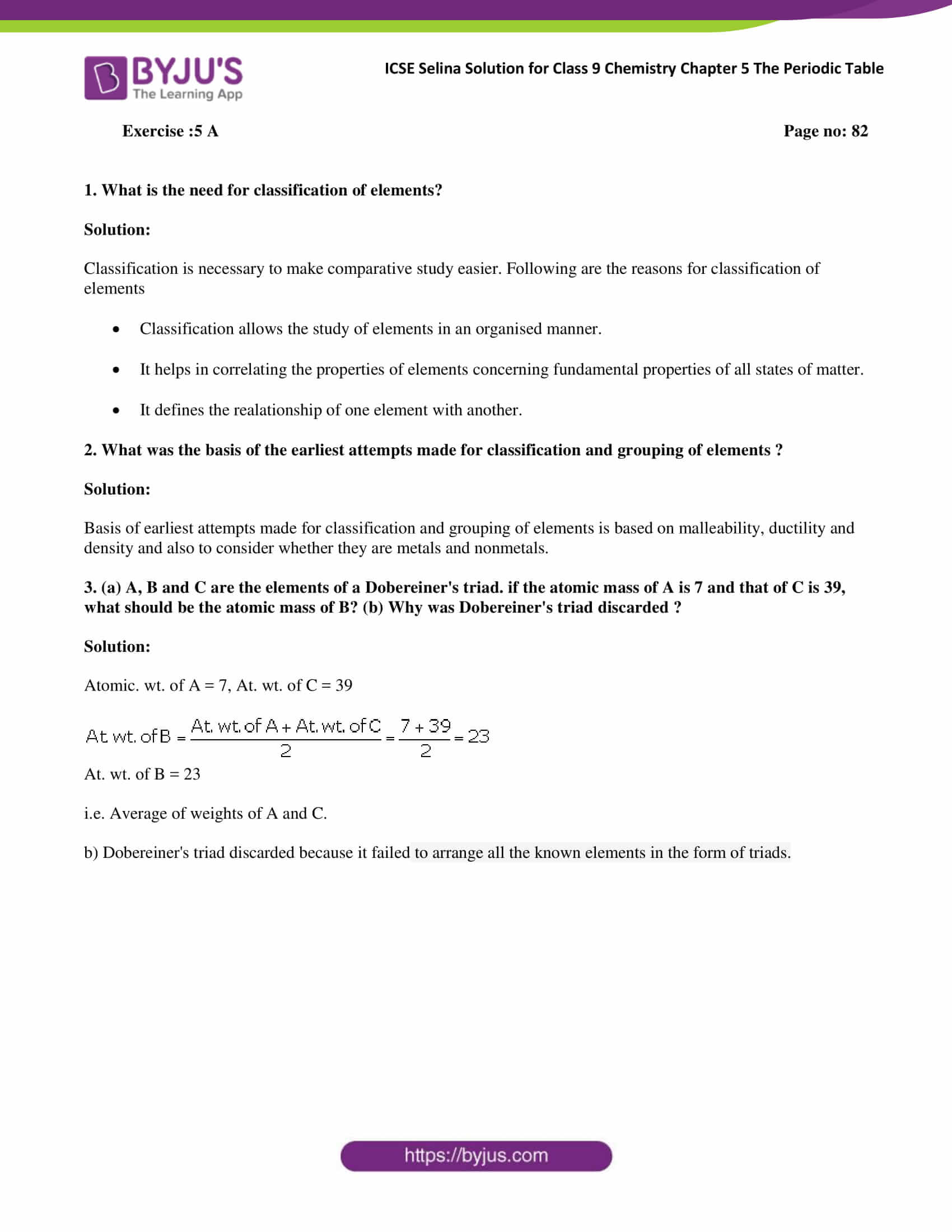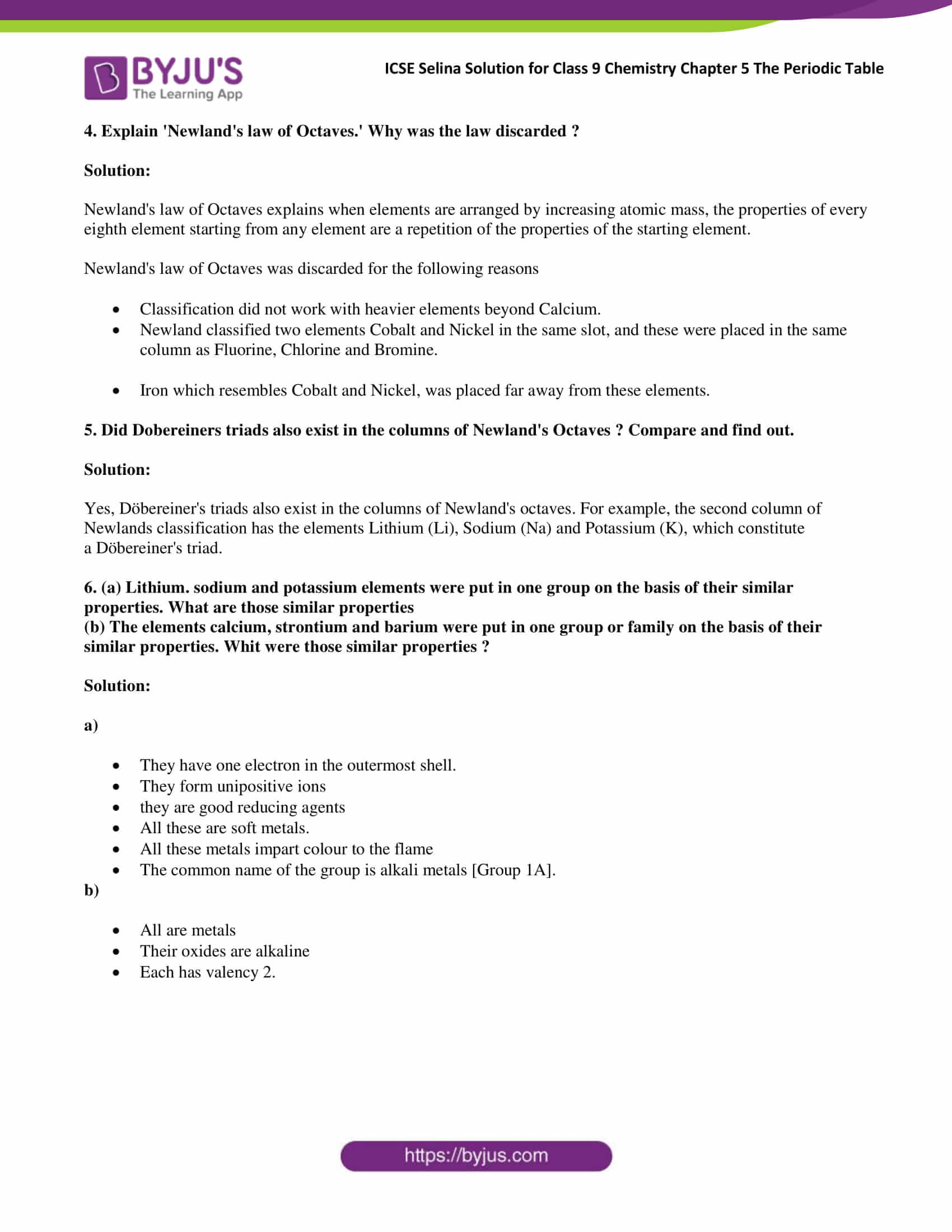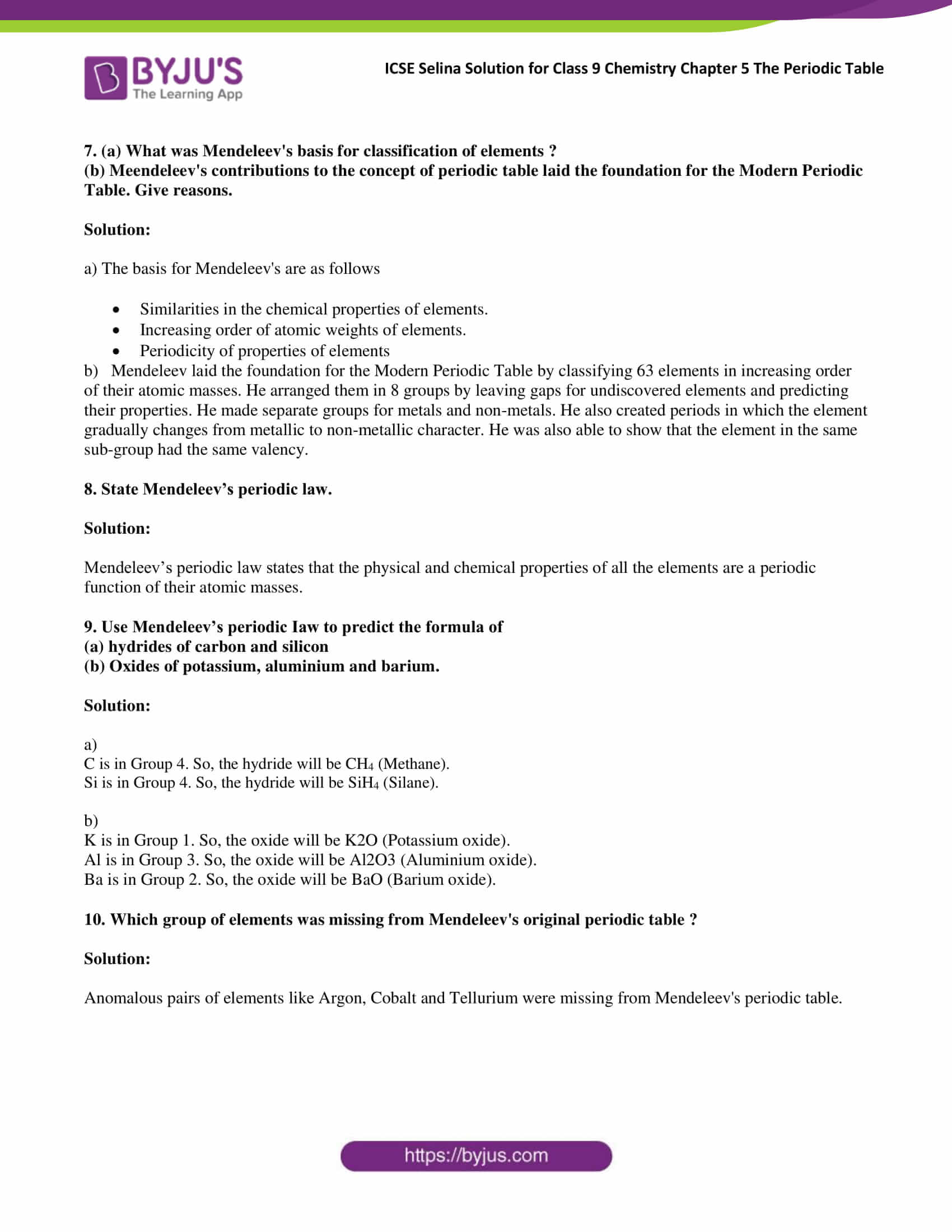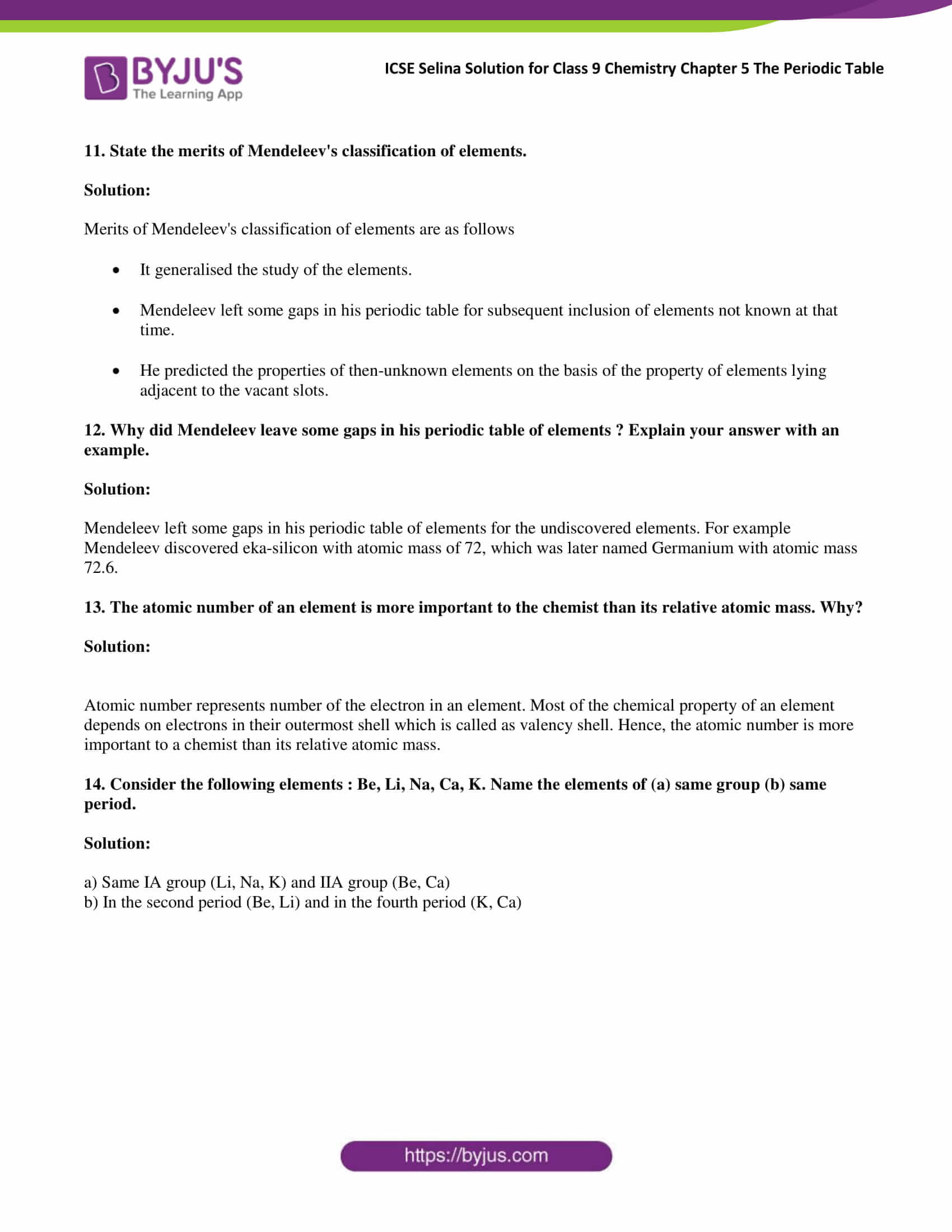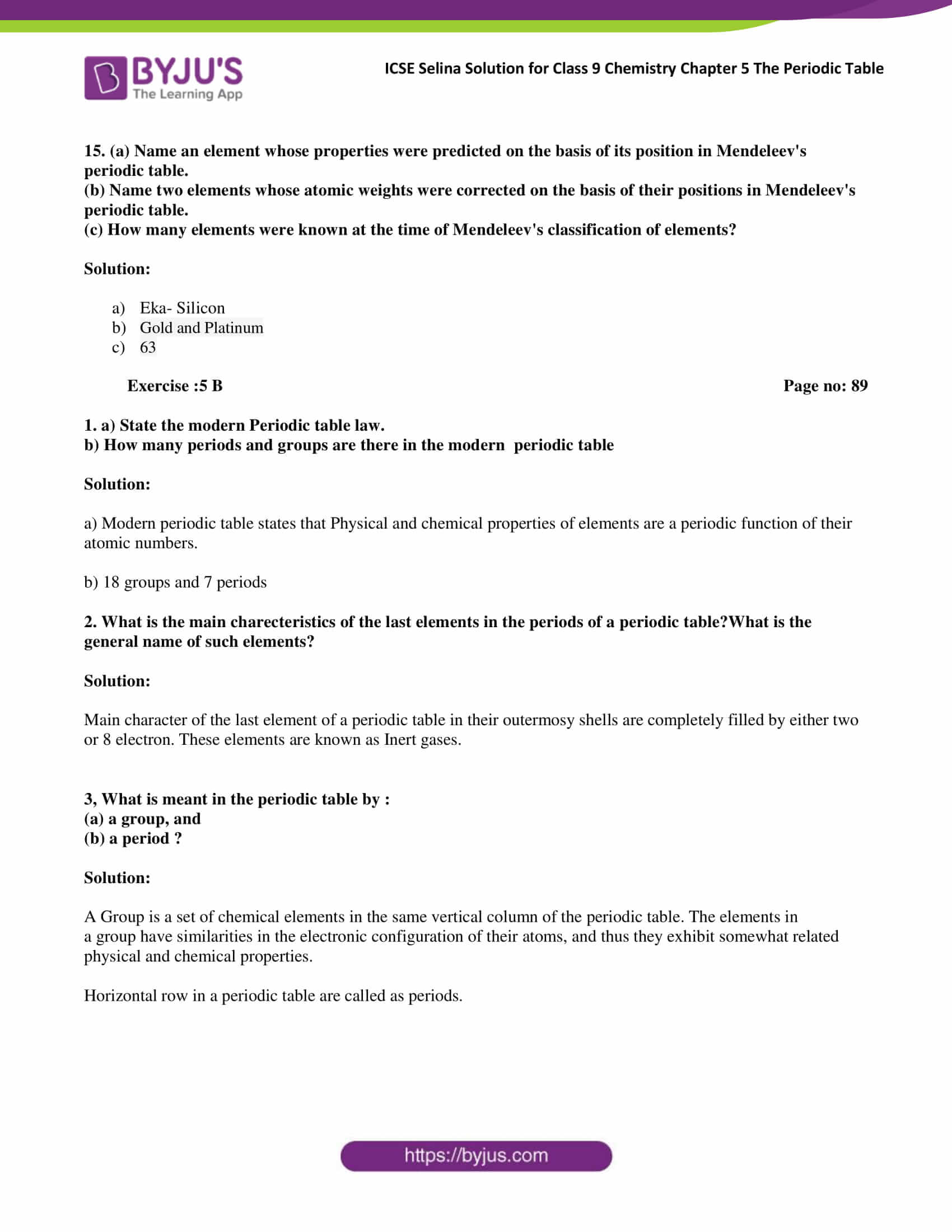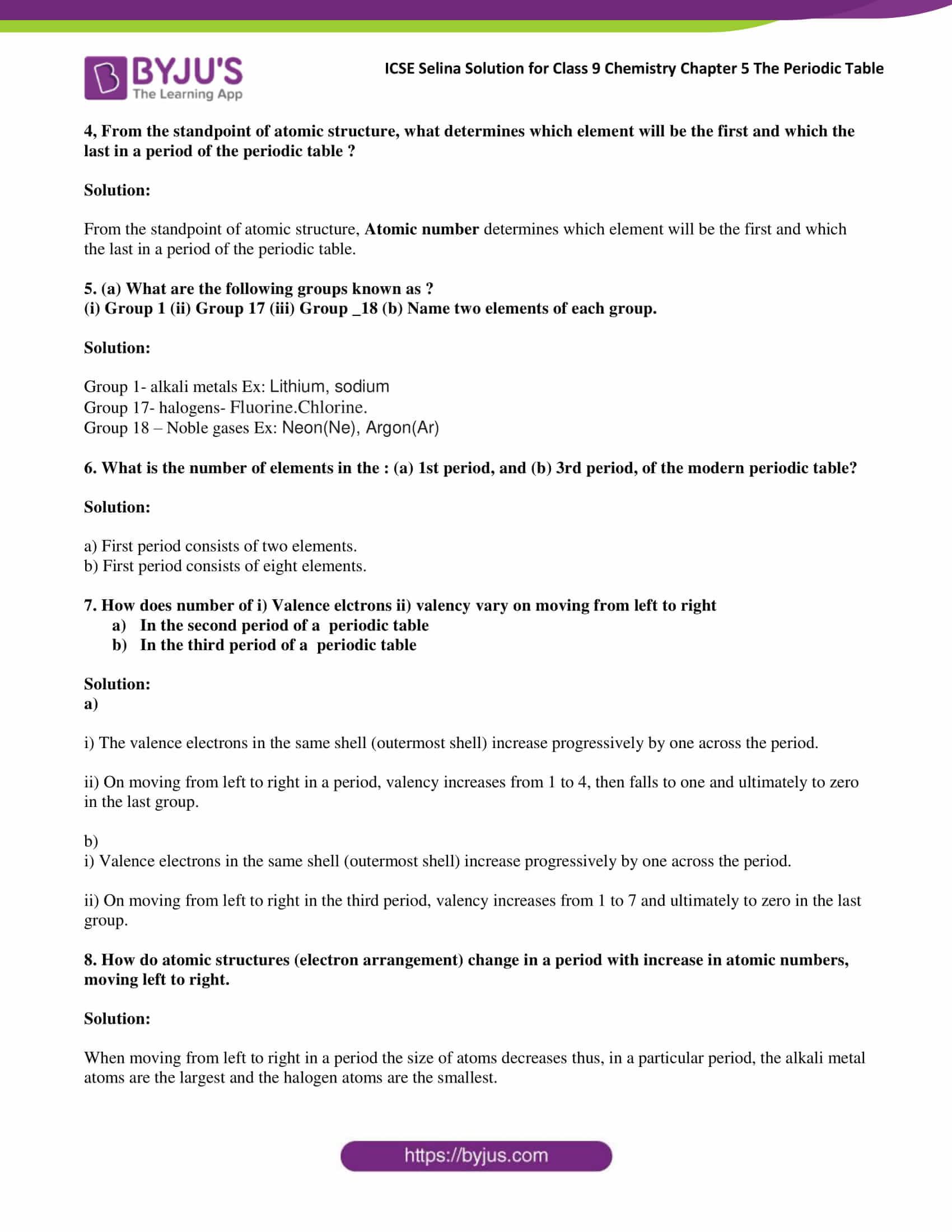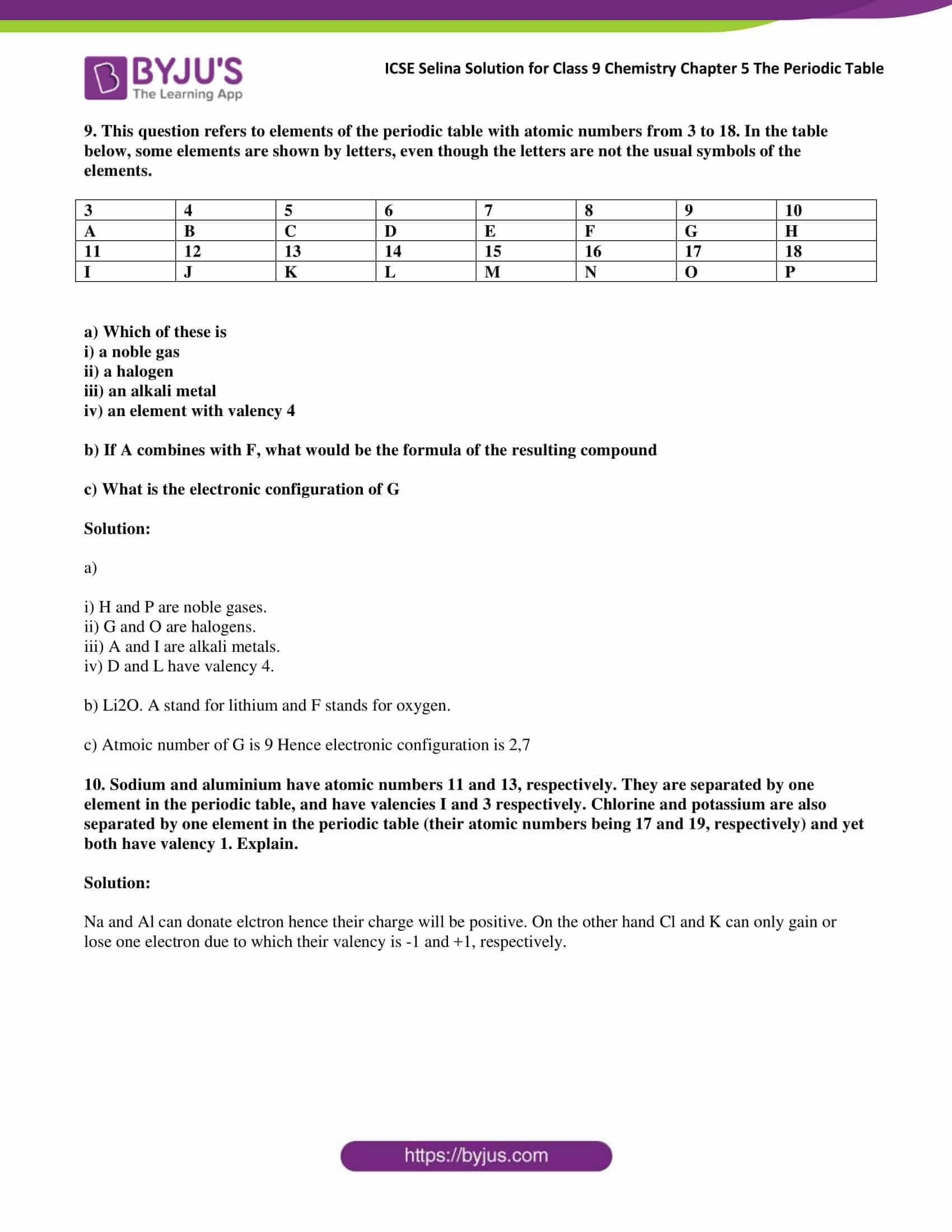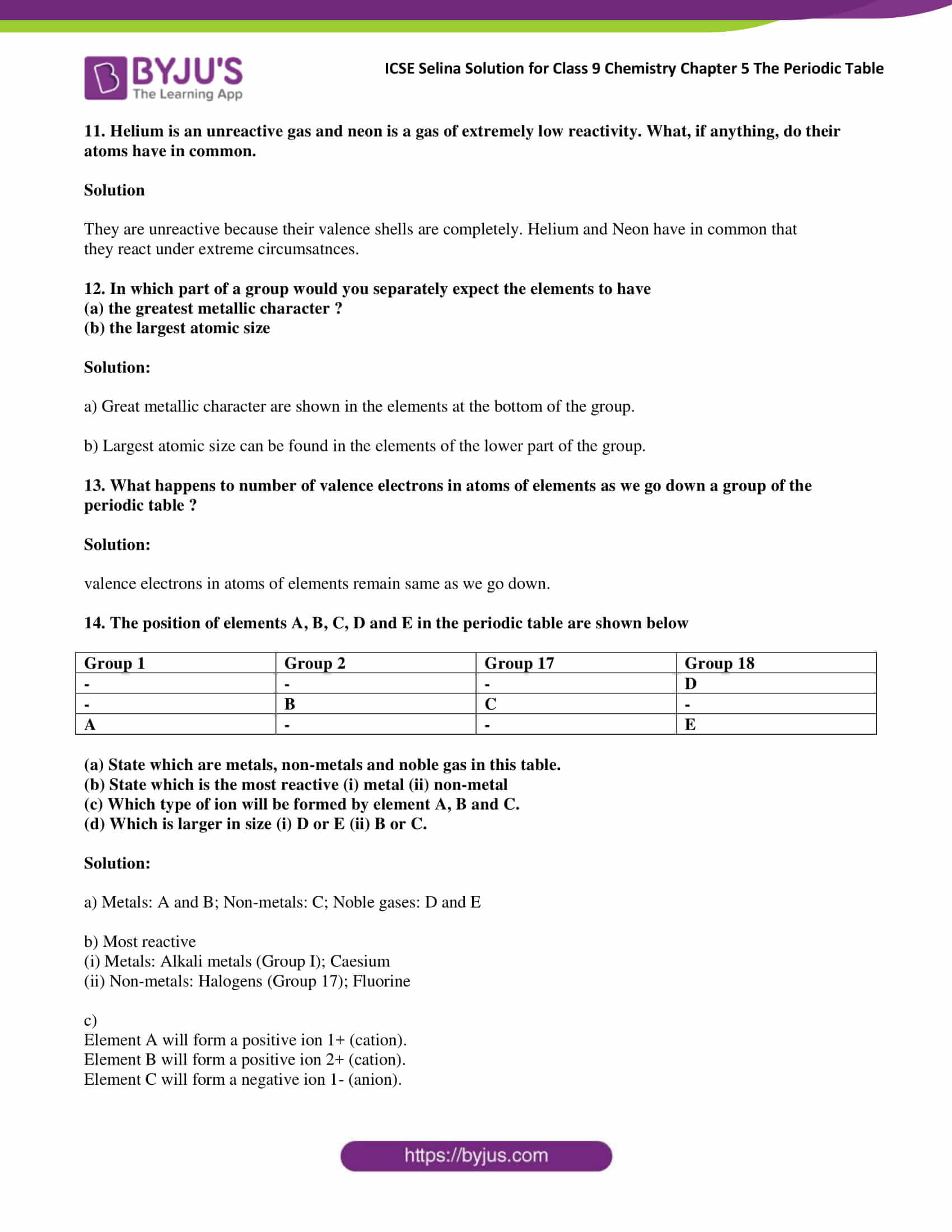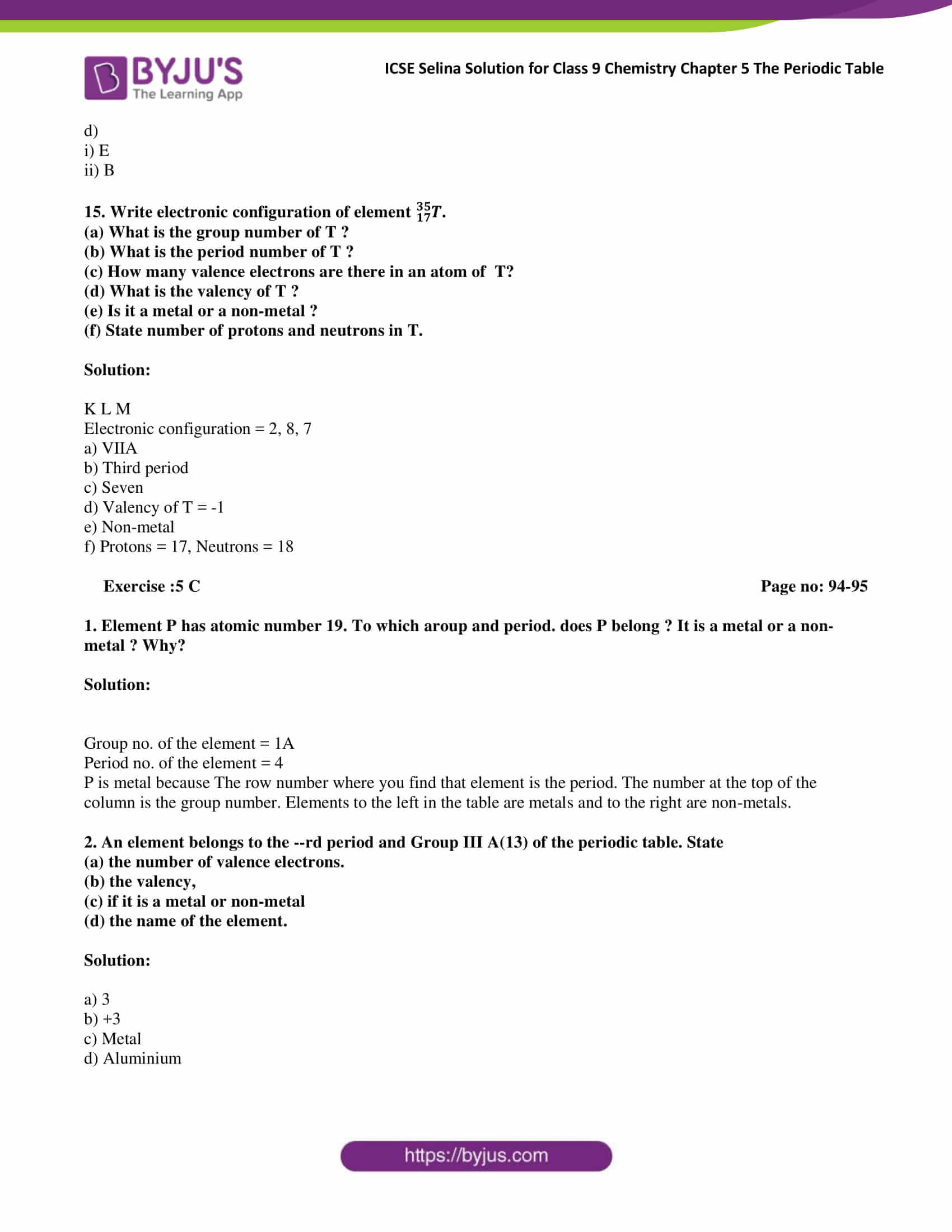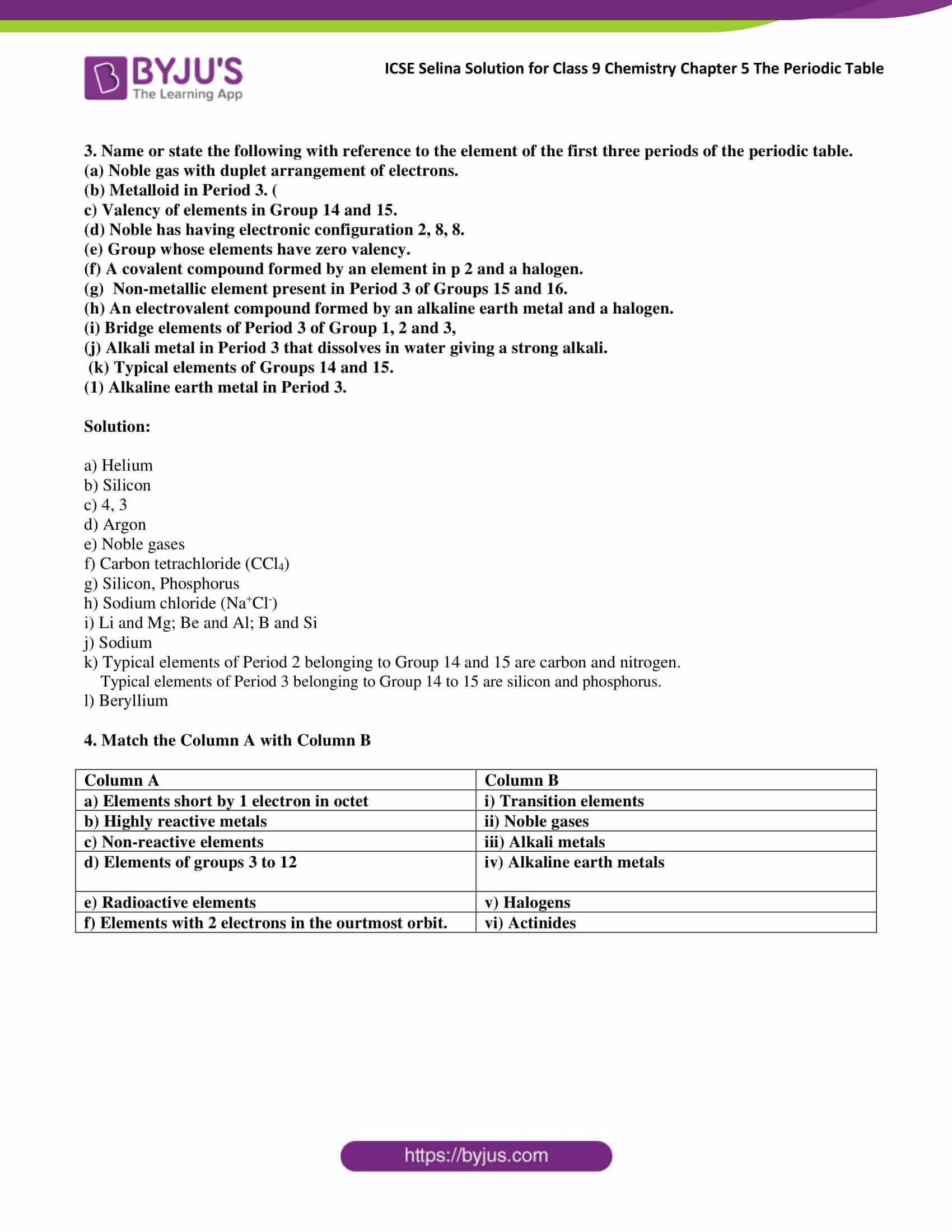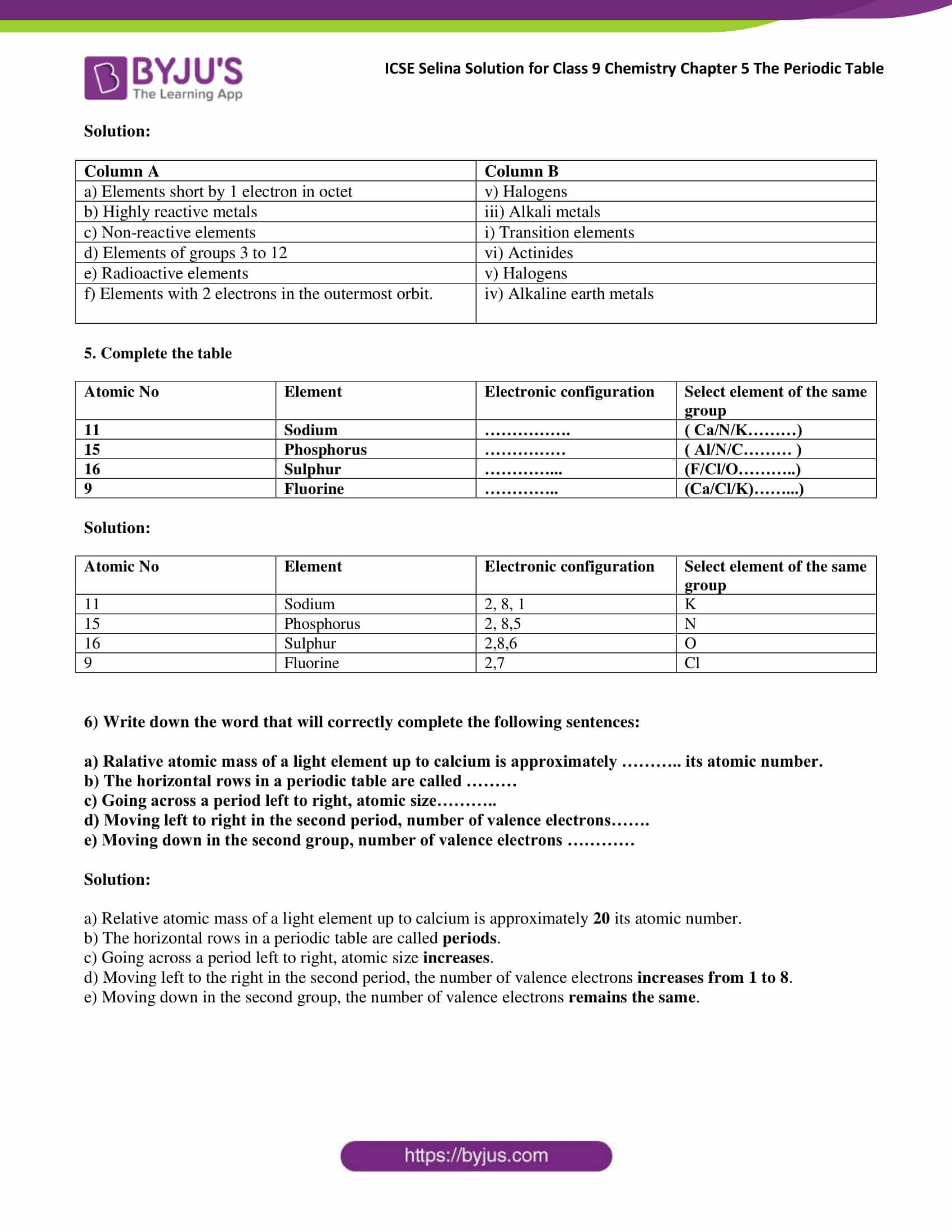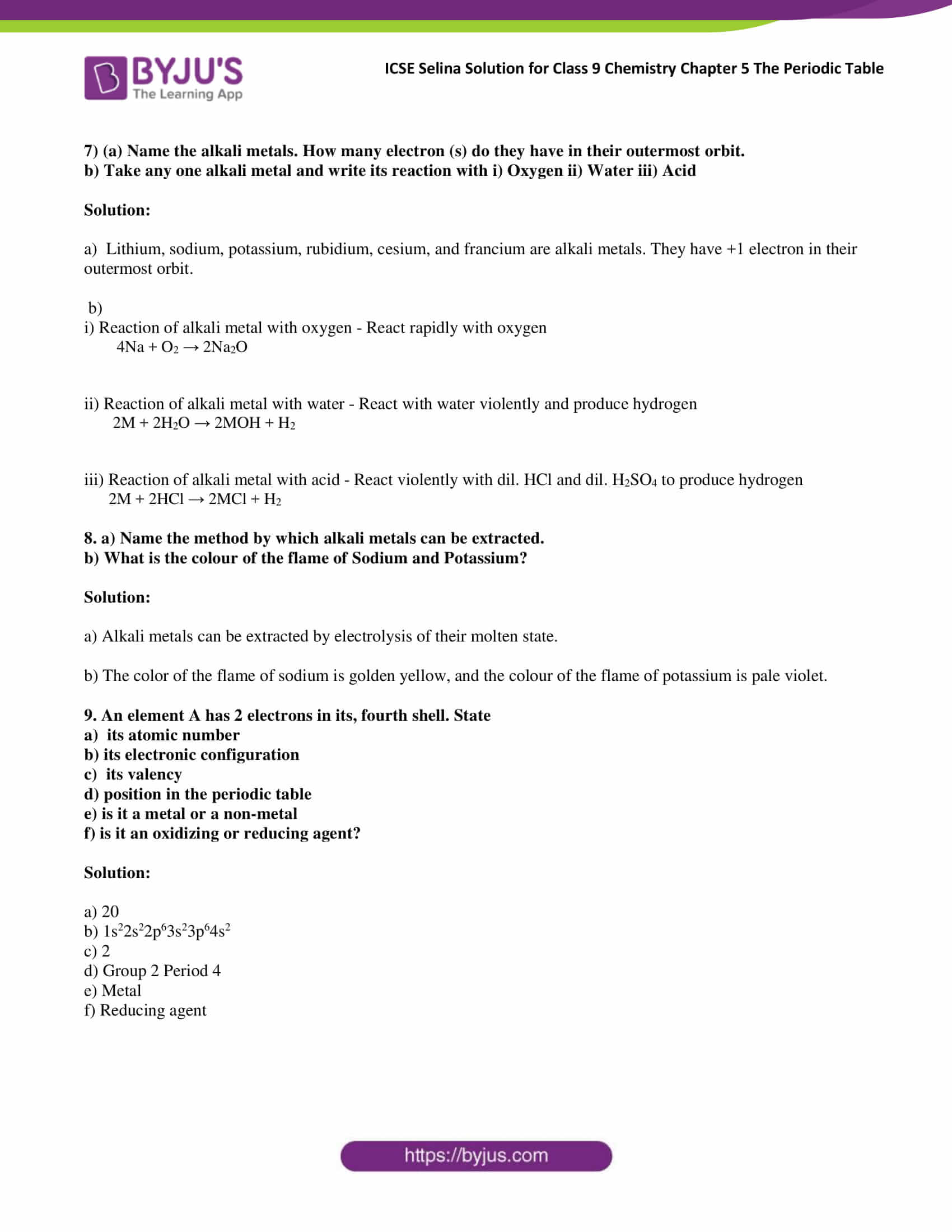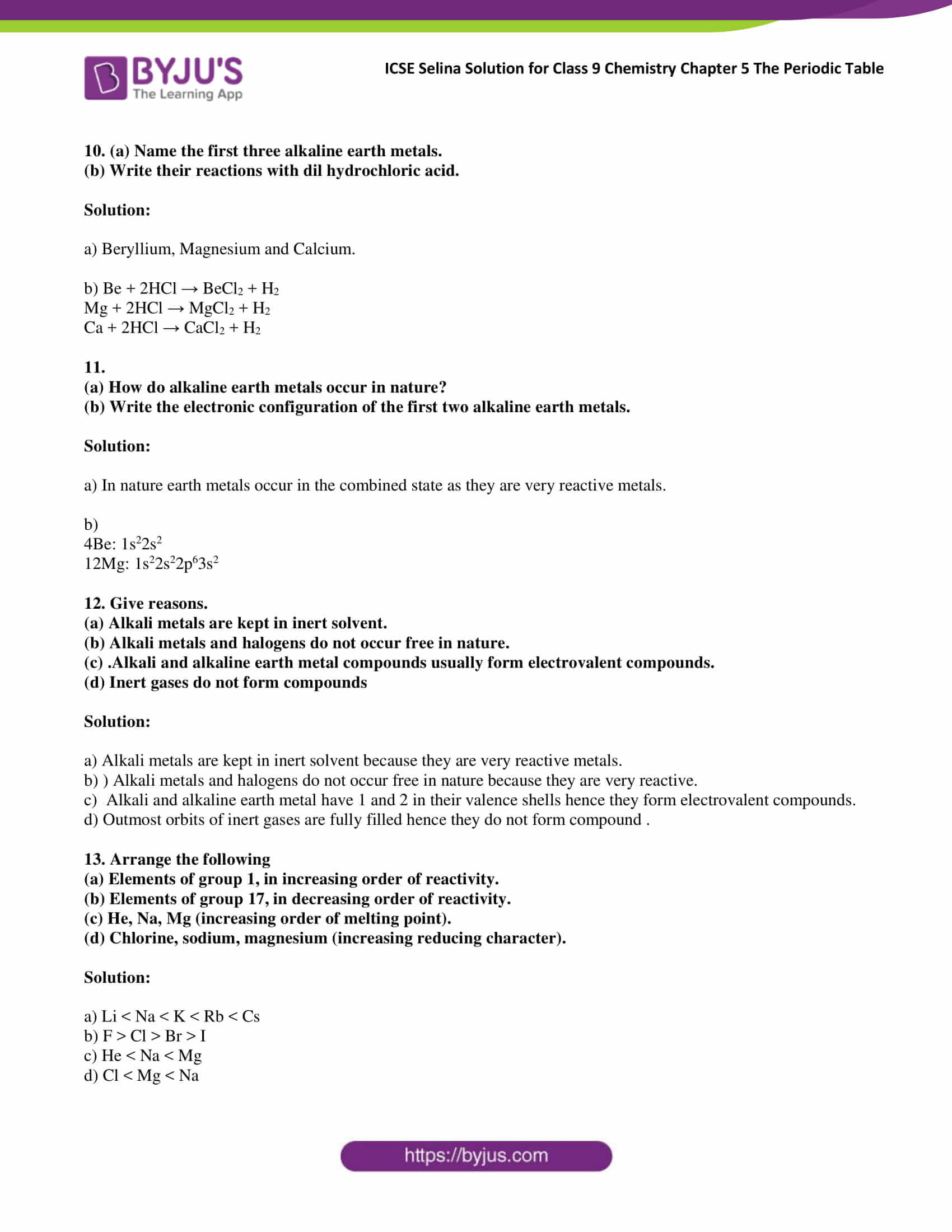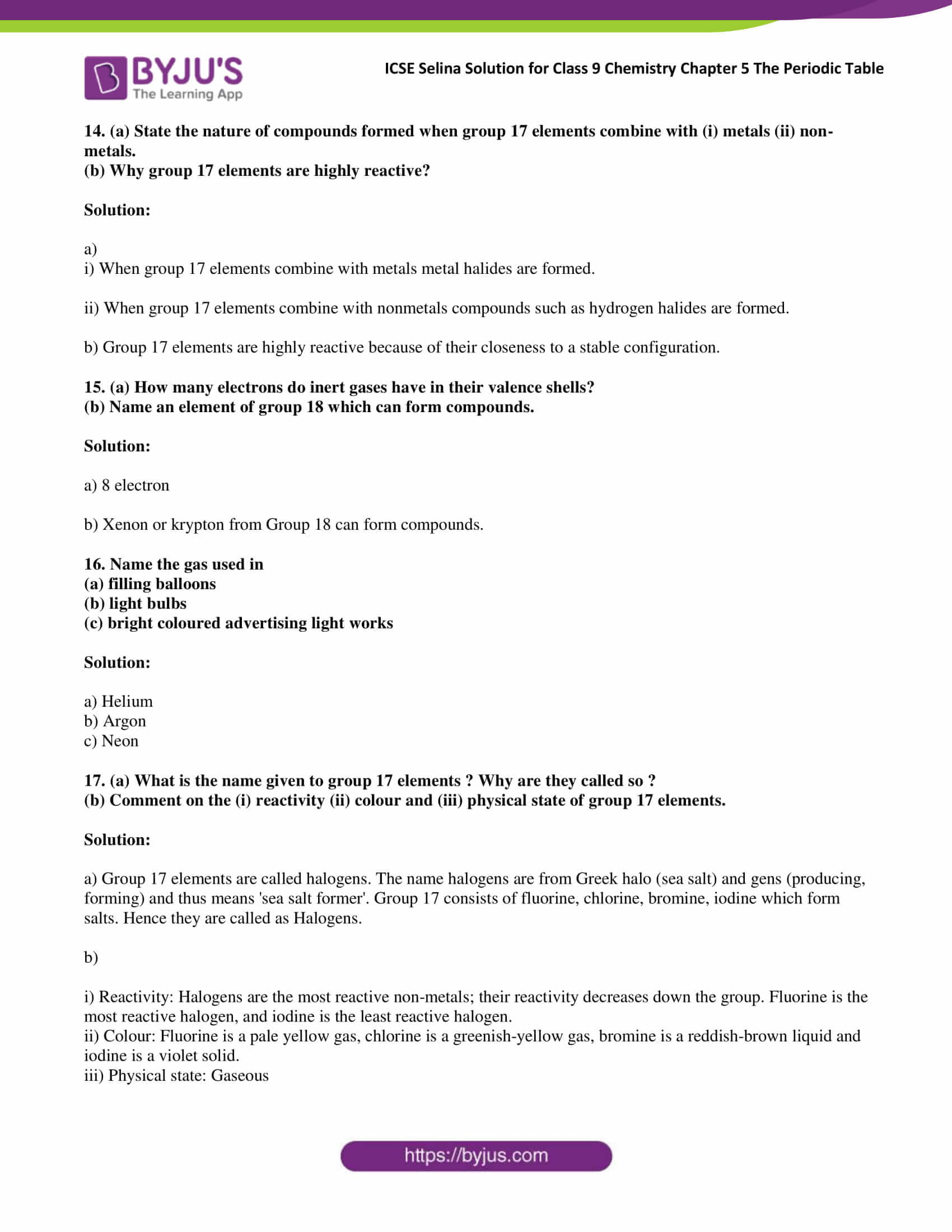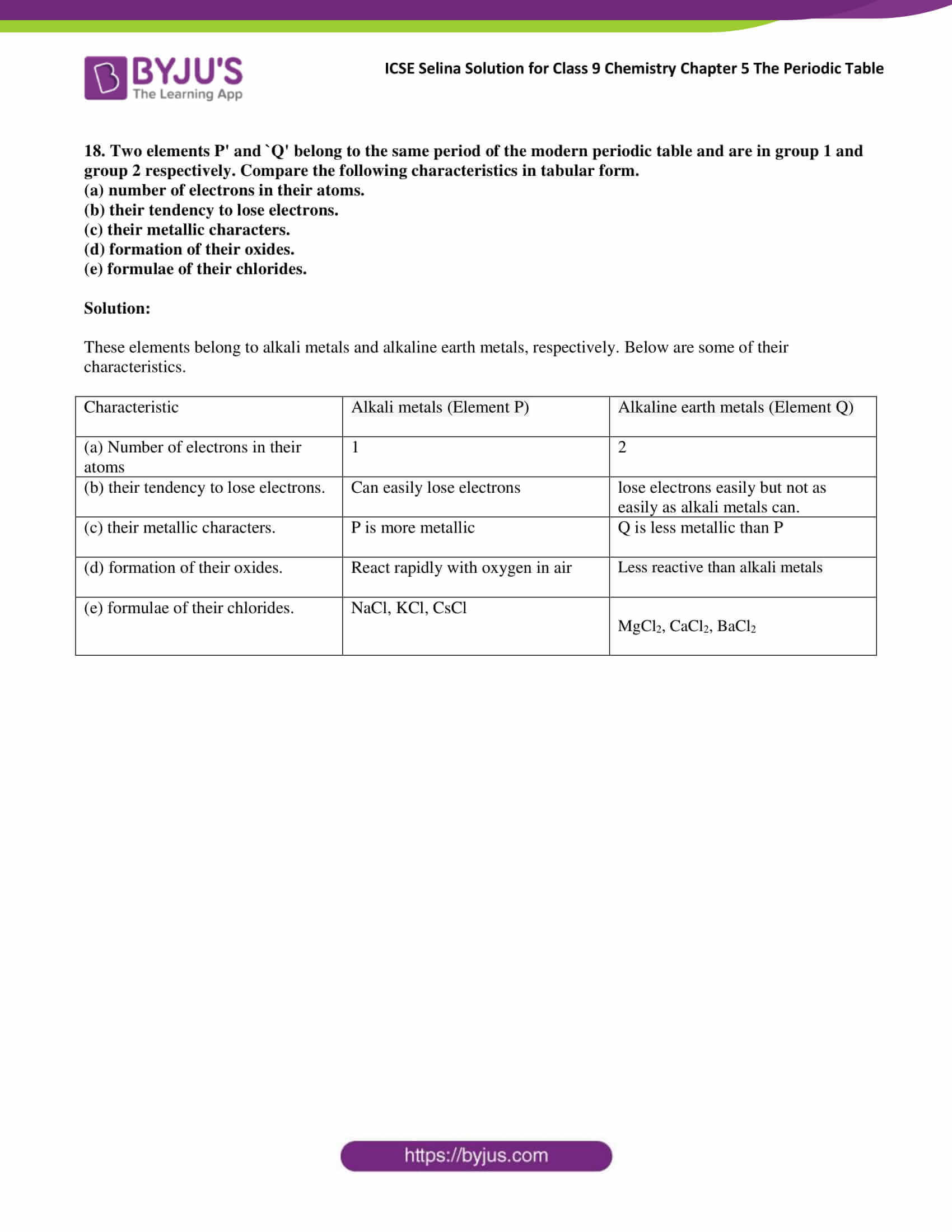### Access Answers of Selina publication ICSE Class 9 Chemistry Chapter 5 The periodic table

Exercise:5 A

1. What is the need for classification of elements?

Solution:

Classification is necessary to make comparative study easier. Following are the reasons for the classification of elements

• Classification allows the study of elements in an organised manner.
• It helps in correlating the properties of elements concerning fundamental properties of all states of matter.
• It defines the relationship of one element with another.

2. What was the basis of the earliest attempts made for classification and grouping of elements?

Solution:

Basis of earliest attempts made for classification and grouping of elements is based on malleability, ductility and density and also to consider whether they are metals and nonmetals.

3. (a) A, B and C are the elements of a Dobereiner’s triad. if the atomic mass of A is 7 and that of C is 39, what should be the atomic mass of B? (b) Why was Dobereiner’s triad discarded?

Solution:

Atomic. wt. of A = 7, At. wt. of C = 39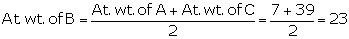At. wt. of B = 23

i.e. Average of weights of A and C.

b) Dobereiner’s triad discarded because it failed to arrange all the known elements in the form of triads.

4. Explain ‘Newland’s law of Octaves.’ Why was the law discarded?

Solution:

Newland’s law of Octaves explains when elements are arranged by increasing atomic mass, the properties of every eighth element starting from any element are a repetition of the properties of the starting element.

Newland’s law of Octaves was discarded for the following reasons

• Classification did not work with heavier elements beyond Calcium.
• Newland classified two elements Cobalt and Nickel in the same slot, and these were placed in the same column as Fluorine, Chlorine and Bromine.
• Iron which resembles Cobalt and Nickel was placed far away from these elements.

5. Did Dobereiners triads also exist in the columns of Newland’s Octaves? Compare and find out.

Solution:

Yes, Döbereiner’s triads also exist in the columns of Newland’s octaves. For example, the second column of Newlands classification has the elements Lithium (Li), Sodium (Na) and Potassium (K), which constitute a Döbereiner’s triad.

6. (a) Lithium. sodium and potassium elements were put in one group on the basis of their similar properties. What are those similar properties

(b) The elements calcium, strontium and barium were put in one group or family on the basis of their similar properties. Whit were those similar properties?

Solution:

a)

• They have one electron in the outermost shell.
• They form unipositive ions
• they are good reducing agents
• All these are soft metals.
• All these metals impart colour to the flame
• The common name of the group is alkali metals [Group 1A].

b)

• All are metals
• Their oxides are alkaline
• Each has valency 2.

7. (a) What was Mendeleev’s basis for classification of elements?

(b) Mendeleev’s contributions to the concept of a periodic table laid the foundation for the Modern Periodic Table. Give reasons.

Solution:

a) The basis for Mendeleev’s are as follows

• Similarities in the chemical properties of elements.
• Increasing order of atomic weights of elements.
• Periodicity of properties of elements

b) Mendeleev laid the foundation for the Modern Periodic Table by classifying 63 elements in increasing order of their atomic masses. He arranged them in 8 groups by leaving gaps for undiscovered elements and predicting their properties. He made separate groups for metals and non-metals. He also created periods in which the element gradually changes from metallic to non-metallic character. He was also able to show that the element in the same sub-group had the same valency.

8. State Mendeleev’s periodic law.

Solution:

Mendeleev’s periodic law states that the physical and chemical properties of all the elements are a periodic function of their atomic masses.

9. Use Mendeleev’s periodic Law to predict the formula of

(a) hydrides of carbon and silicon

(b) Oxides of potassium, aluminium and barium.

Solution:

a)

C is in Group 4. So, the hydride will be CH4 (Methane).

Si is in Group 4. So, the hydride will be SiH4 (Silane).

b)

K is in Group 1. So, the oxide will be K2O (Potassium oxide).

Al is in Group 3. So, the oxide will be Al2O3 (Aluminium oxide).

Ba is in Group 2. So, the oxide will be BaO (Barium oxide).

10. Which group of elements was missing from Mendeleev’s original periodic table ?

Solution:

Anomalous pairs of elements like Argon, Cobalt and Tellurium were missing from Mendeleev’s periodic table.

11. State the merits of Mendeleev’s classification of elements.

Solution:

Merits of Mendeleev’s classification of elements are as follows

• It generalised the study of the elements.
• Mendeleev left some gaps in his periodic table for subsequent inclusion of elements not known at that time.
• He predicted the properties of then-unknown elements on the basis of the property of elements lying adjacent to the vacant slots.

12. Why did Mendeleev leave some gaps in his periodic table of elements? Explain your answer with an example.

Solution:

Mendeleev left some gaps in his periodic table of elements for the undiscovered elements. For example, Mendeleev discovered eka-silicon with an atomic mass of 72, which was later named Germanium with atomic mass 72.6.

13. The atomic number of an element is more important to the chemist than its relative atomic mass. Why?

Solution:

Atomic number represents a number of the electron in an element. Most of the chemical property of an element depends on electrons in their outermost shell which is called a valency shell. Hence, the atomic number is more important to a chemist than its relative atomic mass.

14. Consider the following elements: Be, Li, Na, Ca, K. Name the elements of (a) same group (b) same period.

Solution:

a) Same IA group (Li, Na, K) and IIA group (Be, Ca)

b) In the second period (Be, Li) and in the fourth period (K, Ca)

15. (a) Name an element whose properties were predicted on the basis of its position in Mendeleev’s periodic table.

(b) Name two elements whose atomic weights were corrected on the basis of their positions in Mendeleev’s periodic table.

(c) How many elements were known at the time of Mendeleev’s classification of elements?

Solution:

1. Eka- Silicon
2. Gold and Platinum
3. 63

Exercise:5 B

1. a) State the modern Periodic table law.

b) How many periods and groups are there in the modern periodic table

Solution:

a) The modern periodic table states that Physical and chemical properties of elements are a periodic function of their atomic numbers.

b) 18 groups and 7 periods

2. What are the main characteristics of the last elements in the periods of a periodic table? What is the general name of such elements?

Solution:

The main character of the last element of a periodic table in their outermost shells is completely filled by either two or 8 electrons. These elements are known as Inert gases.

3, What is meant in the periodic table by :

(a) a group, and

(b) a period?

Solution:

A Group is a set of chemical elements in the same vertical column of the periodic table. The elements in a group have similarities in the electronic configuration of their atoms, and thus they exhibit somewhat related physical and chemical properties.

Horizontal row in a periodic table are called as periods.

4, From the standpoint of atomic structure, what determines which element will be the first and which the last in a period of the periodic table ?

Solution:

From the standpoint of atomic structure, Atomic number determines which element will be the first and which the last in a period of the periodic table.

5. (a) What are the following groups known as?

(i) Group 1 (ii) Group 17 (iii) Group _18 (b) Name two elements of each group.

Solution:

Group 1- alkali metals Ex: Lithium, sodium

Group 17- halogens- Fluorine.Chlorine.

Group 18 – Noble gases Ex: Neon(Ne), Argon(Ar)

6. What is the number of elements in the : (a) 1st period, and (b) 3rd period, of the modern periodic table?

Solution:

a) The first period consists of two elements.

b) The first period consists of eight elements.

7. How does the number of i) Valence electrons ii) valency vary on moving from left to right

1. In the second period of a periodic table
2. In the third period of a periodic table

Solution:

a)

i) The valence electrons in the same shell (outermost shell) increase progressively by one across the period.

ii) On moving from left to right in a period, valency increases from 1 to 4, then falls to one and ultimately to zero in the last group.

b)

i) Valence electrons in the same shell (outermost shell) increase progressively by one across the period.

ii) On moving from left to right in the third period, valency increases from 1 to 7 and ultimately to zero in the last group.

8. How do atomic structures (electron arrangement) change in a period with an increase in atomic numbers, moving left to right?

Solution:

When moving from left to right in a period the size of atoms decreases thus, in a particular period, the alkali metal atoms are the largest and the halogen atoms are the smallest.

9. This question refers to elements of the periodic table with atomic numbers from 3 to 18. In the table below, some elements are shown by letters, even though the letters are not the usual symbols of the elements.

 3 4 5 6 7 8 9 10 A B C D E F G H 11 12 13 14 15 16 17 18 I J K L M N O P

a) Which of these is

i) a noble gas

ii) a halogen

iii) an alkali metal

iv) an element with valency 4

b) If A combines with F, what would be the formula of the resulting compound

c) What is the electronic configuration of G

Solution:

a)

i) H and P are noble gases.

ii) G and O are halogens.

iii) A and I are alkali metals.

iv) D and L have valency 4.

b) Li2O. A stand for lithium and F stands for oxygen.

c) The atomic number of G is 9 Hence electronic configuration is 2,7

10. Sodium and aluminium have atomic numbers 11 and 13, respectively. They are separated by one element in the periodic table and have valencies I and 3 respectively. Chlorine and potassium are also separated by one element in the periodic table (their atomic numbers being 17 and 19, respectively) and yet both have valency 1. Explain.

Solution:

Na and Al can donate electron hence their charge will be positive. On the other hand, Cl and K can only gain or lose one electron due to which their valency is -1 and +1, respectively.

11. Helium is an unreactive gas and neon is a gas of extremely low reactivity. What, if anything, do their atoms have in common.

Solution

They are unreactive because their valence shells are complete. Helium and Neon have in common that they react under extreme circumstances.

12. In which part of a group would you separately expect the elements to have

(a) the greatest metallic character?

(b) the largest atomic size

Solution:

a) Great metallic character is shown in the elements at the bottom of the group.

b) Largest atomic size can be found in the elements of the lower part of the group.

13. What happens to a number of valence electrons in atoms of elements as we go down a group of the periodic table?

Solution:

valence electrons in atoms of elements remain the same as we go down.

14. The position of elements A, B, C, D and E in the periodic table are shown below

 Group 1 Group 2 Group 17 Group 18 – – – D – B C – A – – E

(a) State which is metals, non-metals and noble gas in this table.

(b) State which is the most reactive (i) metal (ii) non-metal

(c) Which type of ion will be formed by element A, B and C.

(d) Which is larger in size (i) D or E (ii) B or C.

Solution:

a) Metals: A and B; Non-metals: C; Noble gases: D and E

b) Most reactive

(i) Metals: Alkali metals (Group I); Caesium

(ii) Non-metals: Halogens (Group 17); Fluorine

c)

Element A will form a positive ion 1+ (cation).

Element B will form a positive ion 2+ (cation).

Element C will form a negative ion 1- (anion).

d)

i) E

ii) B

15. Write the electronic configuration of the element .

(a) What is the group number of T?

(b) What is the period number of T?

(c) How many valence electrons are there in an atom of T?

(d) What is the valency of T?

(e) Is it a metal or a non-metal?

(f) State number of protons and neutrons in T.

Solution:

K L M

Electronic configuration = 2, 8, 7

a) VIIA

b) Third period

c) Seven

d) Valency of T = -1

e) Non-metal

f) Protons = 17, Neutrons = 18

Exercise:5 C

1. Element P has atomic number 19. To which group and period. does P belong? It is a metal or a non- metal? Why?

Solution:

Group no. of the element = 1A

Period no. of the element = 4

P is metal because The row number where you find that element is the period. The number at the top of the column is the group number. Elements to the left in the table are metals and to the right are non-metals.

2. An element belongs to the –rd period and Group III A(13) of the periodic table. State

(a) the number of valence electrons.

(b) the valency,

(c) if it is a metal or non-metal

(d) the name of the element.

Solution:

a) 3

b) +3

c) Metal

d) Aluminium

3. Name or state the following with reference to the element of the first three periods of the periodic table.

(a) Noble gas with duplet arrangement of electrons.

(b) Metalloid in Period 3. (

c) Valency of elements in Group 14 and 15.

(d) Noble has having electronic configuration 2, 8, 8.

(e) Group whose elements have zero valency.

(f) A covalent compound formed by an element in p 2 and a halogen.

(g) Non-metallic element present in Period 3 of Groups 15 and 16.

(h) An electrovalent compound formed by an alkaline earth metal and a halogen.

(i) Bridge elements of Period 3 of Group 1, 2 and 3,

(j) Alkali metal in Period 3 that dissolves in the water giving a strong alkali.

(k) Typical elements of Groups 14 and 15.

(1) Alkaline earth metal in Period 3.

Solution:

a) Helium

b) Silicon

c) 4, 3

d) Argon

e) Noble gases

f) Carbon tetrachloride (CCl4)

g) Silicon, Phosphorus

h) Sodium chloride (Na+Cl)

i) Li and Mg; Be and Al; B and Si

j) Sodium

k) Typical elements of Period 2 belonging to Group 14 and 15 are carbon and nitrogen.

Typical elements of Period 3 belonging to Group 14 to 15 are silicon and phosphorus.

l) Beryllium

4. Match the Column A with Column B

 Column A Column B a) Elements short by 1 electron in octet i) Transition elements b) Highly reactive metals ii) Noble gases c) Non-reactive elements iii) Alkali metals d) Elements of groups 3 to 12 iv) Alkaline earth metals e) Radioactive elements v) Halogens f) Elements with 2 electrons in the outermost orbit. vi) Actinides

Solution:

 Column A Column B a) Elements short by 1 electron in octet v) Halogens b) Highly reactive metals iii) Alkali metals c) Non-reactive elements i) Transition elements d) Elements of groups 3 to 12 vi) Actinides e) Radioactive elements v) Halogens f) Elements with 2 electrons in the outermost orbit. iv) Alkaline earth metals

5. Complete the table

 Atomic No Element Electronic configuration A select element of the same group 11 Sodium ……………. ( Ca/N/K………) 15 Phosphorus …………… ( Al/N/C……… ) 16 Sulphur …………… (F/Cl/O………..) 9 Fluorine ………….. (Ca/Cl/K)………)

Solution:

 Atomic No Element Electronic configuration Select element of the same group 11 Sodium 2, 8, 1 K 15 Phosphorus 2, 8,5 N 16 Sulphur 2,8,6 O 9 Fluorine 2,7 Cl

6) Write down the word that will correctly complete the following sentences:

a) The relative atomic mass of a light element up to calcium is approximately ……….. its atomic number.

b) The horizontal rows in a periodic table are called ………

c) Going across a period left to right, atomic size………..

d) Moving left to right in the second period, the number of valence electrons…….

e) Moving down in the second group, the number of valence electrons …………

Solution:

a) The relative atomic mass of a light element up to calcium is approximately 20 its atomic number.

b) The horizontal rows in a periodic table are called periods.

c) Going across a period left to right, atomic size increases.

d) Moving left to the right in the second period, the number of valence electrons increases from 1 to 8.

e) Moving down in the second group, the number of valence electrons remains the same.

7) (a) Name the alkali metals. How many electrons (s) do they have in their outermost orbit.

b) Take any one alkali metal and write its reaction with i) Oxygen ii) Water iii) Acid

Solution:

a)  Lithium, sodium, potassium, rubidium, caesium, and francium are alkali metals. They have +1 electron in their outermost orbit.

b)

i) The reaction of an alkali metal with oxygen – React rapidly with oxygen

4Na + O2 → 2Na2O

ii) The reaction of an alkali metal with water – React with water violently and produce hydrogen

2M + 2H2O → 2MOH + H2

iii) The reaction of an alkali metal with acid – React violently with dil. HCl and dil. H2SO4 to produce hydrogen

2M + 2HCl → 2MCl + H2

8. a) Name the method by which alkali metals can be extracted.

b) What is the colour of the flame of Sodium and Potassium?

Solution:

a) Alkali metals can be extracted by electrolysis of their molten state.

b) The colour of the flame of sodium is golden yellow, and the colour of the flame of potassium is pale violet.

9. An element A has 2 electrons in its, fourth shell. State

a) its atomic number

b) its electronic configuration

c) its valency

d) position in the periodic table

e) is it a metal or a non-metal

f) is it an oxidizing or reducing agent?

Solution:

a) 20

b) 1s22s22p63s23p64s2

c) 2

d) Group 2 Period 4

e) Metal

f) Reducing agent

10. (a) Name the first three alkaline earth metals.

(b) Write their reactions with dil hydrochloric acid.

Solution:

a) Beryllium, Magnesium and Calcium.

b) Be + 2HCl → BeCl2 + H2

Mg + 2HCl → MgCl2 + H2

Ca + 2HCl → CaCl2 + H2

11.

(a) How do alkaline earth metals occur in nature?

(b) Write the electronic configuration of the first two alkaline earth metals.

Solution:

a) In nature, earth metals occur in the combined state as they are very reactive metals.

b)

4Be: 1s22s2

12Mg: 1s22s22p63s2

12. Give reasons.

(a) Alkali metals are kept in an inert solvent.

(b) Alkali metals and halogens do not occur free in nature.

(c) .Alkali and alkaline earth metal compounds usually form electrovalent compounds.

(d) Inert gases do not form compounds

Solution:

a) Alkali metals are kept in the inert solvent because they are very reactive metals.

b) ) Alkali metals and halogens do not occur free in nature because they are very reactive.

c) Alkali and alkaline earth metal have 1 and 2 in their valence shells hence they form electrovalent compounds.

d) Outmost orbits of inert gases are fully filled hence they do not form the compound .

13. Arrange the following

(a) Elements of group 1, in increasing order of reactivity.

(b) Elements of group 17, in decreasing order of reactivity.

(c) He, Na, Mg (increasing order of melting point).

(d) Chlorine, sodium, magnesium (increasing reducing character).

Solution:

a) Li < Na < K < Rb < Cs

b) F > Cl > Br > I

c) He < Na < Mg

d) Cl < Mg < Na

14. (a) State the nature of compounds formed when group 17 elements combine with (i) metals (ii) non-metals.

(b) Why group 17 elements are highly reactive?

Solution:

a)

i) When group 17 elements combine with metals metal halides are formed.

ii) When group 17 elements combine with nonmetals compounds such as hydrogen halides are formed.

b) Group 17 elements are highly reactive because of their closeness to a stable configuration.

15. (a) How many electrons do inert gases have in their valence shells?

(b) Name an element of group 18 which can form compounds.

Solution:

a) 8 electron

b) Xenon or krypton from Group 18 can form compounds.

16. Name the gas used in

(a) filling balloons

(b) light bulbs

(c) bright coloured advertising light works

Solution:

a) Helium

b) Argon

c) Neon

17. (a) What is the name given to group 17 elements? Why are they called so?

(b) Comment on the (i) reactivity (ii) colour and (iii) physical state of group 17 elements.

Solution:

a) Group 17 elements are called halogens. The name halogens are from Greek halo (sea salt) and gens (producing, forming) and thus means ‘sea salt former’. Group 17 consists of fluorine, chlorine, bromine, iodine which form salts. Hence they are called as Halogens.

b)

i) Reactivity: Halogens are the most reactive non-metals; their reactivity decreases down the group. Fluorine is the most reactive halogen, and iodine is the least reactive halogen.

ii) Colour: Fluorine is a pale yellow gas, chlorine is a greenish-yellow gas, bromine is a reddish-brown liquid and iodine is a violet solid.

iii) Physical state: Gaseous

18. Two elements P’ and `Q’ belong to the same period of the modern periodic table and are in group 1 and group 2 respectively. Compare the following characteristics in tabular form.

(a) the number of electrons in their atoms.

(b) their tendency to lose electrons.

(c) their metallic characters.

(d) formation of their oxides.

(e) formulae of their chlorides.

Solution:

These elements belong to alkali metals and alkaline earth metals, respectively. Below are some of their characteristics.

 Characteristic Alkali metals (Element P) Alkaline earth metals (Element Q) (a) Number of electrons in their atoms 1 2 (b) their tendency to lose electrons. Can easily lose electrons lose electrons easily but not as easily as alkali metals can. (c) their metallic characters. P is more metallic Q is less metallic than P (d) formation of their oxides. React rapidly with oxygen in the air Less reactive than alkali metals (e) formulae of their chlorides. NaCl, KCl, CsCl MgCl2, CaCl2, BaCl2

For BYJU’S provides ICSE Books, ICSE Sample papers, Previous year question papers, Solved papers, and important question of ICSE Syllabus for all the subjects and classes Download BYJU’S Learning App.

## Frequently Asked Questions on The Periodic Table

Q1

### Explain ‘Newland’s law of Octaves?

Newland’s law of Octaves explains when elements are arranged by increasing atomic mass, the properties of every eighth element starting from any element are a repetition of the properties of the starting element.

Q2

### Did Dobereiners triads also exist in the columns of Newland’s Octaves?

Yes, Döbereiner’s triads also exist in the columns of Newland’s octaves. For example, the second column of Newlands classification has the elements Lithium (Li), Sodium (Na) and Potassium (K), which constitute a Döbereiner’s triad.

Q3

### What was Mendeleev’s basis for classification of elements?

The basis for Mendeleev’s are as follows

• Similarities in the chemical properties of elements.
• Increasing order of atomic weights of elements.
• Periodicity of properties of elements
Q4

### State Mendeleev’s periodic law?

Mendeleev’s periodic law states that the physical and chemical properties of all the elements are a periodic function of their atomic masses.

Q5

### State the merits of Mendeleev’s classification of elements?

Merits of Mendeleev’s classification of elements are as follows

• It generalised the study of the elements.
• Mendeleev left some gaps in his periodic table for subsequent inclusion of elements not known at that time.
• He predicted the properties of then-unknown elements on the basis of the property of elements lying adjacent to the vacant slots.
Q6

### State the modern Periodic table law?

The modern periodic table states that Physical and chemical properties of elements are a periodic function of their atomic numbers.

Q7

### Group 1 Known has?

Group 1- alkali metals Ex: Lithium, sodium

Q8

### Group 17 known has?

Group 17- halogens- Fluorine,Chlorine.

Q9

### Group 18 known has?

Group 18 – Noble gases Ex: Neon(Ne), Argon(Ar)